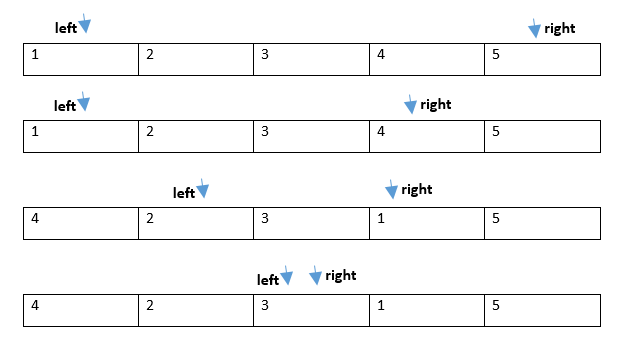# Arrange Even and Odd number such that Odd comes after Even

INPUT: 7  2  4  9  10  11  13  27
OUTPUT: 10  2  4  9  7  11  13  27

### ALGORITHM

TIME COMPLEXITY: O(N)

SPACE COMPLEXITY: O(1)

1. Simply initialize two variables named left and right at starting index and ending index respectively.
2. Loop till left is less than right and do
• If element at index left is odd then check if element at index right is odd or even.
• If the element at index right is odd then simply decrement right variable till we get any even number and right index being greater than left index.
• Else swap the element at left and right index.
• Loop terminates when left equals right.```#include <bits/stdc++.h>
using namespace std;

int main()
{
int arr[] = {4,1,8,9,11,3,77,2};
int N = sizeof(arr)/sizeof(arr);

int left = 0, right =N-1; //left at start index and right at end index
while(left < right) //till left index is less than right index
{
if(arr[left]%2) //if array at left index is odd
{
while((arr[right]%2 ==1) and right > left)	//then loop backwards if element at right index is odd
right --;
swap(arr[left++],arr[right--]);//swap the even and odd elements to bring even element at front and odd at back.
}
else
left++;
}

for(int i=0;i<N;i++)
cout<<arr[i]<<" ";
return 0;
}```

Translate »